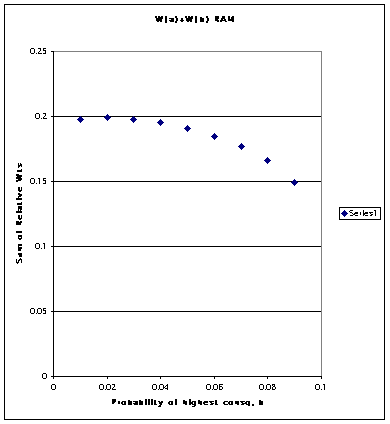### Erratum

In Birnbaum (in press), the following two paragraphs should be deleted:

"Unlike stripped prospect theory, RAM and TAX models do not, however, violate transparent dominance (Birnbaum, 1999a): improving the consequence of a given branch (holding everything else constant) improves a gamble. Similarly, moving probability from a branch with a lower valued consequence to a branch with a higher valued consequence (holding everything else constant) improves the gamble.

"Although they satisfy transparent dominance, both RAM and TAX violate first order stochastic dominance for specially constructed choices (Birnbaum, 1997)."

I have come to the realization that probability monotonicity can be viewed as a combination of coalescing, branch independence, and consequence monotonicity. Therefore, models that violate coalescing or branch independence, as do RAM and TAX, can potentially be made to violate probability monotonicity. If we regard probability monotonicity as "transparent", then RAM and TAX can violate transparent dominance.

Because RAM and TAX satisfy idempotence, they do not have the same types of implausible violations of transparent dominance that were described by Fishburn (1978); nevertheless, they do violate probability monotonicity in certain circumstances, as will be described below.

For example, suppose 0 < z < x < y, and consider the gamble (z, 1 — p — q; x, p; y, q). We can split the branch leading to x as follows: (z, 1 — p — q; x, p-r; x, r; y, q). Next increase the consequence on the (x, r) splinter to y. We now have (z, 1 — p — q; x, p — r; y, r; y, q). Now coalesce the two branches leading to consequence y: (z, 1 — p — q; x, p — r; y, r + q). So, probability monotonicity is implied by these three premises.

This intuition led me to a region in which RAM violates probability monotonicity for three-branch gambles and TAX satisfies it, given their prior parameters. An experiment is currently under way to test these differential predictions of prior TAX and RAM. The paradigm involves a variation of Birnbaum’s recipe for violations of stochastic dominance, illustrated with the following choices:

 ```A: 90 red marbles to win \$96 05 blue marbles to win \$14 05 white marbles to win \$12``` ```B: 85 green marbles to win \$96 05 black marbles to win \$90 10 yellow marbles to win \$12``` ```A: 90 red marbles to win \$96 05 blue marbles to win \$14 05 white marbles to win \$12``` ```B’: 15 green marbles to win \$96 75 black marbles to win \$90 10 yellow marbles to win \$12```

Note that B’ dominates B by probability monotonicity. To create B’ from B, take 75% to win \$96 and decrease it to \$90, and coalesce it with the 5% to win \$90. Clearly, we have made B’ worse than B. Nevertheless, RAM implies that B’ has a higher value than B. To understand why RAM has this implication, realize that the branch weights are a negatively accelerated function of objective probability. This means that the sum of the weights of the two higher branches will depend on how the probability of those branches is split. When the two branches have equal probability, the total weight of these branches will be greater than when one of them has a high value and the other low. The total relative weight of the two upper branches in RAM is plotted below, as a function of the probability of the branch with the highest consequence, where the sum of probability is fixed at 0.10. There are two factors contributing to the violation of probability monotonicity: First, by transferring weight from the highest branch to the middle branch, that probability receives a weight of 2 rather than 1, because the branch weights are 3, 2, and 1 in the RAM model for lowest, middle, and highest branch. Second, as the split deviates from (.05, .05) to (.09, .01), the total relative weight is decreased becausewhere g < 1.### References

Birnbaum, M. H. (in press). Causes of Allais common consequence paradoxes: An experimental dissection. Journal of Mathematical Psychology.

Fishburn, P. C. (1978). On Handa's "New theory of cardinal utility" and the maximization of expected return. Journal of Political Economy, 86(2), 321-324.